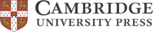## Partial regularisation of the incompressible -rheology for granular flow

T. Barker, J. M. N. T. Gray
• Journal of Fluid Mechanics, August 2017, Cambridge University Press
• DOI: 10.1017/jfm.2017.428

# Partial regularisation of the incompressible μ(I)-rheology for granular flow

In recent years considerable progress has been made in the continuum modelling of granular flows, in particular the μ(I)-rheology, which links the local viscosity in a flow to the strain rate and pressure through the non-dimensional inertial number . This formulation greatly benefits from its similarity to the incompressible Navier–Stokes equations as it allows many existing numerical methods to be used. Unfortunately, this system of equations is ill posed when the inertial number is too high or too low. The consequence of ill posedness is that the growth rate of small perturbations tends to infinity in the high wavenumber limit. Due to this, numerical solutions are grid dependent and cannot be taken as being physically realistic. In this paper changes to the functional form of the μ(I) curve are considered, in order to maximise the range of well-posed inertial numbers, while preserving the overall structure of the equations. It is found that when the inertial number is low there exist curves for which the equations are guaranteed to be well posed. However when the inertial number is very large the equations are found to be ill-posed regardless of the functional dependence of on μ on I. A new curve, which is inspired by the analysis of the governing equations and by experimental data, is proposed here. In order to test this regularised rheology, transient granular flows on inclined planes are studied. It is found that simulations of flows, which show signs of ill-posedness with unregularised models, are numerically stable and match key experimental observations when the regularised model is used. This paper details two-dimensional transient computations of decelerating flows where the inertial number tends to zero, high-speed flows that have large inertial numbers, and flows which develop into granular rollwaves. This is the first time that granular rollwaves have been simulated in two dimensions, which represents a major step towards the simulation of other complex granular flows.

### Why is it important?

Classical Coulomb friction states that tangential forces are proportional to normal forces by a constant of proportionality μ. As 3D generalisations of this idea, the Drucker-Prager and Mohr-Coulomb models were developed to link the shear-stess and pressure (tensorial analogues of tangential and normal forces). Recently another modification has been made which makes μ non-constant and instead dependent on the non-dimensional strain-rate, known as the inertial number I. This dependence on the inertial number is designed to capture the additional dissipation generated when grains move faster relative to one another. The μ(I)-rheology, which results from this change, is a significant step towards modelling many real-world granular flows and allows matching with numerous experimental measurements. However, the dynamic equations which result from this rheology are ill-posed for certain parameters. Ill-posedness says that small perturbations grow unboundedly in the limit that their wavelnegth is infinitesimal. This is particularly problematic for numerical calculations where results will depend on the spatial resolution used and be dominated quickly by these pertubations. The μ(I)-rheolog is ill-posed when the inertial number is both too high and when it is too low. For intermediate values it is re-assuringly well-posed. In this paper, changes are made to the functional form of the μ(I) relation that makes the resultant transient equations well-posed for all inertial numbers below a very large maximal value. This partially-regularised system of equations is able, for the first time, to reliably capture decelerating flows on inclined chutes, granular rollwaves and high-speed steady flows. Such a major breakthrough for the stability and applicability of these models gives great hope that truly complex time-dependent granular flows can be simulated.

### Perspectives

##### Thomas Barker
University of Manchester

Ill-posedness often points to missing physics in a model. Here we find that the equations for the μ(I)-rheology are well-posed for inertial numbers present in many of the experiments that inspired the model. However, as numerous other physical granular flows of practical interest have regions in the ill-posed ranges, a method of regularisation is vital. This use of linear stability analysis has therefore revealed a surprising connection between the mathematical results and physical disparity in continuum modelling. This profound change to the stability of the equations means that they can be solved for slow flows, approaching static material, and for fast flows, which can develop into granular rollwaves. Such developments, therefore, represent a major step towards the general prediction of complex granular flows. Furthermore, due to the direct analogy between these equations and the incompressible Navier--Stokes equations, many existing numerical methods may be easily modified to solve these partially-regularised μ(I) equations. Closer matching with certain experimental results may however require the inclusion of additional physical effects. One simple addition is to allow the local solids volume fraction φ to vary. This leads to compressible μ(I)-equations which can be made well-posed given certain criteria on the dissipative terms (Barker, Schaeffer, Shearer & Gray Proc. R. Soc. A 2017 and Heyman, Delannay, Tabuteau & Valance J. Fluid Mech. 2017). Another set of well-posed equations can be derived for shallow flows (Baker, Barker & Gray J. Fluid Mech. 2016). The advantage of depth-averaged models is their low computational cost when compared to full 2D/3D simulations, this suggests a more immediate applicability to large complex flows in nature.

http://dx.doi.org/10.1017/jfm.2017.428

The following have contributed to this page: Thomas Barker and Professor John M N T GrayIn partnership with: